### The Formulae

This program will be used by observers for scheduling their observations with LUCI. The following fixed parameters
• telescope (transmission, light-collecting area, reflectivity, ...)
• transmission of the instruments (entrance window, lenses, ...)
• quantum efficiency (QE) of the detector
and user-defined parameters
• object (geometry, spectrum, magnitude)
• camera (scale)
• filter
• grating, slit width (spectroscopic mode)
• atmospheric conditions (airmass, water vapor, sky background, seeing)
• adaptive optics (is loop closed, Strehl ratio)
• exposure parameters (detector integration time, total exposure time)
• signal-to-noise ratio
are used to calculate two auxiliary values:
 E = Tatm . Ttel . Tinst . Tfilt . QE (1) F = F0 . 10-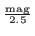(2)

 F0 : flux density for Vega at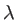= 550 nm F0 = 3.56 . 10-11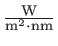[FLUX95] mag : magnitude of the object QE : quantum efficiency of the detector T atm : transmission of the atmosphere T tel : transmission of the telescope T inst : transmission of the instrument (without any filter and grating) T filt : transmission of the filter used

The number of photons that are detected per second can be calculated with (1), (2) and the following formula [ESO-HP]:

 Observing mode point source extended source Imaging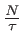=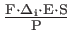=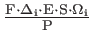Spectroscopy=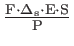=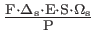N : number of photons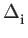: filter band width P : energy of one photon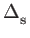: spectral resolution S : light-collecting area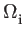: scale in imaging mode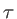: exposure time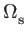: scale in spectroscopy mode

In the near-infrared regime the SNR for the exposure time DIT is given by the formula:

 SNRDIT =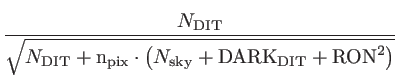(3)

 DARK DIT : dark current for 1 DIT n pix : number of integration pixels1 Nsky : sky signal for 1 DIT RON : readout noise SNRDIT : signal-to-noise ratio for 1 DIT

In imaging mode the user will be asked for the signal-to-noise ratio for the exposure time( SNR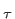). The ETC calculates the necessary exposure time to achieve this SNR(see also formula 3):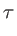= NDIT . DIT   and   SNR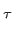= SNRDIT .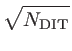(4)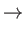=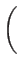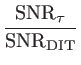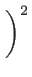. DIT (5): total exposure time DIT : detector integration time NDIT : number of detector integrations SNR: signal-to-noise ratio for exposure time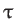SNRDIT : signal-to-noise ratio for one DIT SNR : signal-to-noise ratio for an exposure time of 1sec

Andre Germeroth 2016-11-04